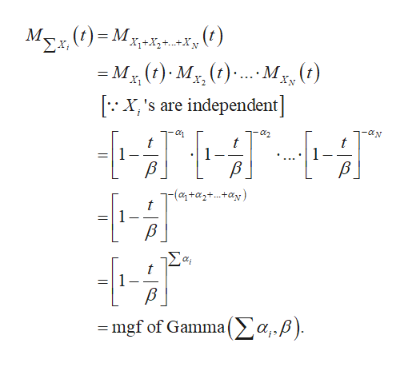# Suppose that Xi ∼ Gamma(αi , β) independently for i = 1, . . . , N. The mgf(moment generating function) of Xiis MXi(t) = (1 − (t/β) )−αi . (a)Use the mgf of Xi to derive the mgf of ∑i=1 Xi .Use the mgf of Xi to derive the mgf of ∑i=1 Xi

Question
27 views

Suppose that Xi ∼ Gamma(αi , β) independently for i = 1, . . . , N. The mgf(moment generating function) of Xiis MXi(t) = (1 − (t/β) )−αi . (a)Use the mgf of Xi to derive the mgf of ∑i=1 Xi .

Use the mgf of Xi to derive the mgf of ∑i=1 Xi

check_circle

Step 1

Introduction:

It is given that Xi’s (i = 1, 2, …, N) are independent Gamma (αi, β) random variables with mgf as follows:

Step 2

Derivation:

The mgf of the sum of all Xi’s, that is, of Σ Xi is calculated as follows:help_outlineImage TranscriptioncloseM()M+Xx ,(e) =Mx (t) My (t).. .M(t) X, are independent] B -(a+a2+..ay = mgf of Gamma (>a.B) fullscreen
Step 3

Conclusion:

The above derivation shows that Σ Xi has the Gamma (Σ α...

### Want to see the full answer?

See Solution

#### Want to see this answer and more?

Solutions are written by subject experts who are available 24/7. Questions are typically answered within 1 hour.*

See Solution
*Response times may vary by subject and question.
Tagged in

### Other